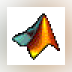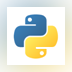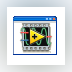### MATLAB R2012a

MATLAB is a programming environment for algorithm development, data analysis, visualization, and numerical computation.### MATLAB R2015a (32-bit)

MATLAB (Matrix Laboratory) is a simulation package for engineering applications.### MATLAB Compiler Runtime

The MATLAB Runtime is a standalone set of shared libraries that enables the execution of compiled MATLAB applications ...### MATLAB Student

MATLAB is the language of technical computing at leading engineering and science companies and the standard software at more than 5000 universities worldwide.### MATLAB R2016a

MATLAB (Matrix Laboratory) is a mathematical and scientific computational tool.### Komodo Edit

Komodo Edit is a text editor that can be used to develop programs in various languages.

... Java, JavaScript, Matlab, C#, C , VHDL, MXML ...### Image Analyzer

Advanced image editing, enhancement and analysis software. The program contains both most image enhancement features ...

... , HIPS and Matlab files Morphological ...### Python - spyder

Spyder (previously known as Pydee) is a free open-source Python development environment providing MATLAB-like features ...

... Pydee) is a free open-source ... environment providing MATLAB-like features ...### Frame 3D

2 on 1 vote

FRAME3DD is a program for the static and dynamic structural analysis of two- and three-dimensional frames and trusses with elastic and geometric stiffness.

... prescribed displacements - Matlab and spreadsheet ...### Scilab

Scilab is a mathematical computing program similar to MATLAB.### ECG Viewer

A Matlab GUI for reviewing, processing, and annotating electrocardiogram (ECG) data files.### V-REP PRO EDU

V-REP is a robot simulator with an integrated development environment.

... , Java, Lua, Matlab, Octave or ...### Engauge Digitizer

This open source, digitizing software converts an image file showing a graph or map, into numbers.

... , gnuplot, gnumeric, MATLAB and Mathematica ... source and free courtesy of ...### FreeMat

FreeMat is an environment for rapid engineering and scientific processing.

... such as MATLAB from Mathworks ...